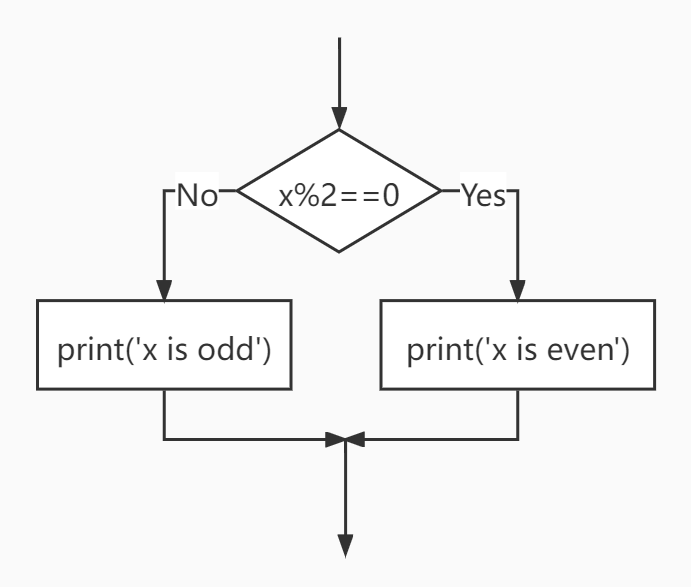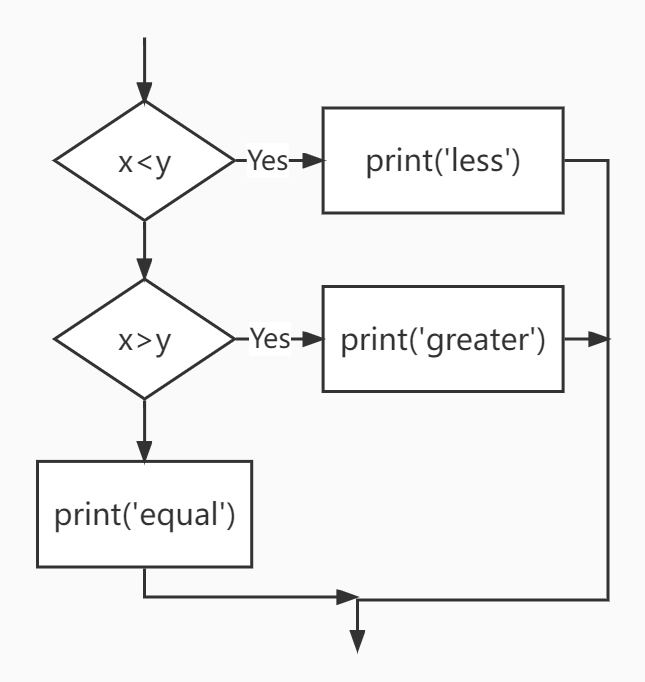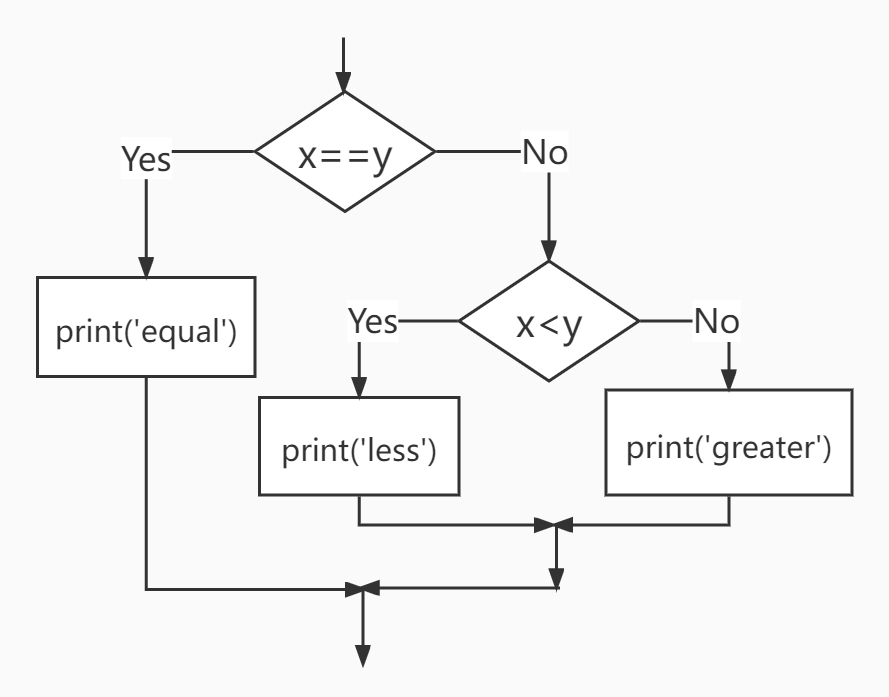# Python Programming

## 2.1 Boolean Expressions (布尔表达式)

A boolean expression is an expression that is either true or false.


print(5==5)
print(5==6)


True
False


Note that here we use a double equal sign $==$.

True and False are special values that belong to the class bool, not strings.


print(type(True))
print(type(False))


bool
bool


There are other ways of comparison.


x != y # x is not equal to y
x > y # x is greater than y
x < y # x is less than y
x >= y # x is greater than or equal to y
x <= y # x is less than or equal to y


#### Logical operators: and, or, not


print(True and True)
print(True and False)
print(False and False)
print(5 > 3 and 3 > 1)


True
False
False
True


print(True or True)
print(True or False)
print(False or False)
print(5 > 3 or 1 > 3)


True
True
False
True


print(not True)
print(not False)
print(not 1>2)



False
True
True


Any nonzero number is interpreted as "true".


print(17 and True)


True


#### Short-circuit evaluation

• Python evaluates the expression from left to right.
• If there are multiple expressions, Python evaluates them one by one.
• If there is nothing to be gained by evaluating the rest of a logical expression, it stops its evaluation.

x = 6
y = 2
print(x >= 2 and (x/y) > 2)


True


x = 1
y = 0
print(x >= 2 and (x/y) > 2)


False


x = 6
y = 0
print(x >= 2 and (x/y) > 2)


Traceback (most recent call last):
File "<stdin>", line 1, in <module>
ZeroDivisionError: division by zero

• While this may seem like a fine point, the short-circuit behavior leads to a clever technique called the guardian pattern.

x = 1
y = 0
print(x >= 2 and y!= 0 and (x/y) > 2)


False


x = 6
y = 0
print(x >= 2 and y!= 0 and (x/y) > 2)
#y!= 0 acts as a guard.


False


x = 6
y = 0
print(x >= 2 and (x/y) > 2 and y != 0)



Traceback (most recent call last):
File "<stdin>", line 1, in <module>
ZeroDivisionError: division by zero


## 2.2 Conditional Executionx = 10
if x > 0:
print('x is positive')

• The boolean expression after the if statement is called the condition.

• We end the if statement with a colon character :

• If the logical condition is true, then the indented statement gets executed.

• The indent is important!
• There is no limit on the number of statements, but there must be at least one.

• If there is no statement, you can use pass statement, which does nothing.

• 
if x < 0:
pass
# need to handle negative values!


#### Alternative Executionx=10
if x%2 == 0 :
print('x is even')
else :
print('x is odd')


#### Chained Conditionalsx=5
y=10
if x < y:
print('x is less than y')
elif x > y:
print('x is greater than y')
else:
print('x and y are equal')

• elif is an abbreviation of “else if.”

• No limit on the number of elif statements. If there is an else clause, it has to be at the end, but there does not have to be one.

• Each condition is checked in order. If the first is false, the next is checked. If one of them is true, then it executes, and the statement ends.

#### Nested Conditionalsif x == y:
print('x and y are equal')
else:
if x < y:
print('x is less than y')
else:
print('x is greater than y')

• Nested conditionals are difficult to read. In general, it is a good idea to avoid them when you can.

#### Try and Except


inp = input('Enter Fahrenheit Temperature: ')
fahr = float(inp)
cel = (fahr - 32.0) * 5.0 / 9.0
print(cel)


Enter Fahrenheit Temperature:72
22.22222222222222

Enter Fahrenheit Temperature:fred


Traceback (most recent call last):
File "fahren.py", line 2, in <module>
fahr = float(inp)
ValueError: could not convert string to float: 'fred'


The idea of try and except is that you know that some sequence of instruction(s) may have a problem and you want to add some statements to be executed if an error occurs. These extra statements (the except block) are ignored if there is no error.


inp = input('Enter Fahrenheit Temperature:')
try:
fahr = float(inp)
cel = (fahr - 32.0) * 5.0 / 9.0
print(cel)
except:


Enter Fahrenheit Temperature:72
22.22222222222222

Enter Fahrenheit Temperature:fred


#### Example 1: BMI Calculator v1.0


print("BMI指数计算器\n")
inp_1 = input('请输入您的体重(kg):\n')
inp_2 = input('请输入您的身高(cm):\n')
weight = float(inp_1)
height = float(inp_2)


BMI = weight/(height/100)**2
if BMI<18.5:
print("您的体型偏瘦")
elif BMI<24 and BMI>=18.5:
print("您的体型正常")
elif BMI<28 and BMI>=24:
print("您的体型偏胖")
elif BMI<32 and BMI>=28:
print("您的体型肥胖")
elif BMI>=32:
print("您的体型过于肥胖")


#### Example 2: A Simple Game

• What are the key elements of a game?
• Making a choice and feedback.

## 2.3 Lists (1): Index

A list is a sequence of values.


[10, 20, 30, 40]
['apple', 'banana', 'orange']
['spam', 2.0, 5, [10, 20]]


A list that contains no elements is called an empty list; you can create one with empty brackets, [].


>>> cheeses = ['Cheddar', 'Edam', 'Gouda']
>>> numbers = [17, 123]
>>> empty = [] #[] is false
>>> print(cheeses, numbers, empty)
['Cheddar', 'Edam', 'Gouda'] [17, 123] []

• Lists are ordered collections, so you can access any element in a list by telling Python the position, or index, of the item desired.
• Python considers the first item in a list to be at position 0, not position 1.
• 
>>> cheeses = ['Cheddar', 'Edam', 'Gouda']
>>> print(cheeses)
Cheddar

• When we ask for a single item from a list, Python returns just that element without square brackets or quotation marks.
• By asking for the item at index -1, Python always returns the last item in the list:
• 
>>> cheeses = ['Cheddar', 'Edam', 'Gouda']
>>> print(cheeses[-1])
Gouda

>>> print(cheeses[-2])
Edam

• You can change the order of items in a list or reassign an item in a list.
• 
>>> numbers = [17, 123]
>>> numbers = 5
>>> print(numbers)
[17, 5]

• The + operator concatenates lists:
• 
>>> a = [1, 2, 3]
>>> b = [4, 5, 6]
>>> c = a + b
>>> print(c)
[1, 2, 3, 4, 5, 6]


>>>  * 4
[0, 0, 0, 0]

>>> [1, 2, 3] * 3
[1, 2, 3, 1, 2, 3, 1, 2, 3]


#### List slices


>>> t = ['a', 'b', 'c', 'd', 'e', 'f']
>>> t[1:3]
['b', 'c']

>>> t[:4]
['a', 'b', 'c', 'd']

>>> t[3:]
['d', 'e', 'f']

• The first slice starts at 1, and ends at 2. The slice does not contain the 3rd element.
• 
>>> t[:]
['a', 'b', 'c', 'd', 'e', 'f']


>>> t = ['a', 'b', 'c', 'd', 'e', 'f']
>>> t[1:3] = ['x', 'y']
>>> print(t)
['a', 'x', 'y', 'd', 'e', 'f']


## 2.4 Lists (2): Adding and Deleting

• append adds a new element to the end of a list:

• 
>>> t = ['a', 'b', 'c']
>>> t.append('d')
>>> print(t)
['a', 'b', 'c', 'd']

• extend takes a list as an argument and appends all of the elements:

• 
>>> t1 = ['a', 'b', 'c']
>>> t2 = ['d', 'e']
>>> t1.extend(t2)
>>> print(t1)
['a', 'b', 'c', 'd', 'e']

• insert adds a new element at any position in your list

• 
>>> t = ['a', 'b', 'c']
>>> t.insert(1, 'd')
>>> print(t)
['a', 'd', 'b', 'c']

• What is Method? (方法) Is it the same with function? (函数)
• The return value of the above three methods is None. What is None in Python?

• 
>>> t = ['a', 'b', 'c']
>>> x = t.insert(1, 'd')
>>> print(t)
['a', 'd', 'b', 'c']

>>> print(x)
None

• The None keyword is used to define a null value, or no value at all. None is not the same as 0, False, or an empty string. None is a datatype of its own (NoneType) and only None can be None.

### Deleting elements

• There are several ways to delete elements from a list. If you know the index of the element you want, you can use pop

• 
>>> t = ['a', 'b', 'c']
>>> x = t.pop(1)
>>> print(t)
['a', 'c']

>>> print(x)
b

• pop modifies the list and returns the element that was removed. If you don't provide an index, it deletes and returns the last element.

• 
>>> t = ['a', 'b', 'c']
>>> x = t.pop()
>>> print(t)
['a', 'b']

>>> print(x)
c

• If you don't need the removed value, you can use the del operator

• 
>>> t = ['a', 'b', 'c']
>>> del t
>>> print(t)
['a', 'c']

>>> t = ['a', 'b', 'c', 'd', 'e', 'f']
>>> del t[1:5]
>>> print(t)
['a', 'f']

• If you know the element you want to remove (but not the index), you can use remove

• 
>>> t = ['a', 'b', 'c']
>>> t.remove('b')
>>> print(t)
['a', 'c']

• The return value from remove is None. But, you can assign the element which you want to remove to a variable so that you can use it next time.

• There is a possibility that the value appears more than once in the list, but the remove() method deletes only the first occurrence.

• Sometimes you not only want to modify the list but also want to save the original one. You can create a copy.
• 
>>> t1 = ['a', 'b', 'c']
>>> t2 = t1[:]
>>> t1.remove('b')
>>> print(t1)
['a', 'c']

>>> print(t2)
['a', 'b', 'c']


## 2.5 Lists (3): Organizing a list

• sort arranges the elements of the list from low to high:

• 
>>> t = ['d', 'c', 'e', 'b', 'a']
>>> t.sort()
>>> print(t)
['a', 'b', 'c', 'd', 'e']


>>> t = ['d', 'c', 'e', 'b', 'a']
>>> t.sort(reverse=True)
>>> print(t)
['e', 'd', 'c', 'b', 'a']

• Most list methods modify the list and return None.

• By .sort(), the order of the list is permanently changed.

• To maintain the original order of a list but present it in a sorted order, you can use the sorted() function.

• 
>>> t = ['d', 'c', 'e', 'b', 'a']
>>> print(sorted(t))
['a', 'b', 'c', 'd', 'e']

>>> print(t)
['d', 'c', 'e', 'b', 'a']

>>> t = ['d', 'c', 'e', 'b', 'a']
>>> t=sorted(t)
>>> print(t)
['a', 'b', 'c', 'd', 'e']

• To reverse the original order of a list, you can use the reverse() method.

• 
>>> t = ['d', 'c', 'e', 'b', 'a']
>>> t.reverse()
>>> print(t)
['a', 'b', 'e', 'c', 'd']


• The reverse() method changes the order of a list permanently, but you can revert to the original order anytime by applying reverse() to the same list a second time.

• You can quickly find the length of a list by using the len() function.

• 
>>> s = ['python', 'java', ['asp', 'php'], 'scheme']
>>> len(s)
4

>>> L = []
>>> len(L)
0


>>> s = [3, 8, 10, 7]
>>> sum(s)
28

>>> min(s)
3

>>> max(s)
10


>>> x = ['banana', 'jack', 'jessica']
>>> min(x)
banana

>>> max(x)
jessica

>>> sum(x)

TypeError: unsupported operand type(s)
for +: 'int' and 'str'


### Lists: Summary

• The element can be number, string, list, and empty [].

• Features: Ordered, Mutable, Repeatable

• The indices start at 0. Index -1 means the last item.

• The item can be changed by reassign a new item. Two lists can be added together. list slice :

• .append(), .extend(), .insert()

.pop(), del, .remove()

.sort(), sorted(), .reverse(), len(), sum(), max(), min()

## Summary

• Boolean Expressions
• Conditional Execution
• Lists
• Reading: Python for every body Chapter 3, 8.1-8.7